# WShit

 博客园  :: 首页  :: 新随笔  :: 联系 :: 订阅:: 管理

# 一、PTA实验作业

## 题目1：填充方阵

### 1.本题PTA提交列表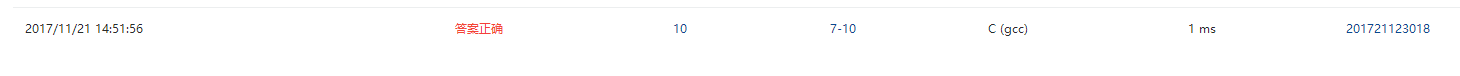### 2.设计思路

定义array储存矩阵元素
for r=1 to 7
array[r][r]=array[r][8-r]=1;
for c=1 to 7
如果c>r&&c<8-r，array[r][c]=2
如果c<r&&c<8-r，array[r][c]=3
如果c>r&&c>8-r，array[r][c]=4
如果c>8-r&&c<r，array[r][c]=5
输出array[r][c]
end for
换行
end


### 3.代码截图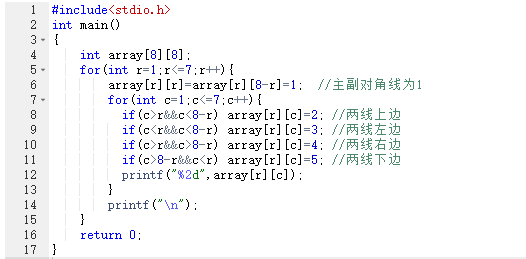• 无出现问题.

## 题目2：简易连连看

### 1. 本题PTA提交列表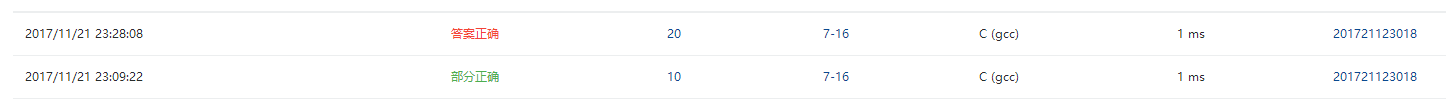### 2.设计思路

定义(void)output(int  array[],int n)用于输出盘面
for r=1 to n
for c=1 to n-1
输出array[r][c]
end
输出array[r][n]
换行
end

main()
(int)定义k表示连线次数,chance=3表示可出错三次,x1,x2,y1,y2表示坐标,clear=0表示已清除字数数,array储存矩阵元素.

for r=1 to 2n
for c=1 to 2n
输入array[r][c].
吸收多余字符  end  end

for i=1 to k&&chance!=0
输入坐标x1,y1,x2,y2
如果array[x1][y1]==array[x2][y2]&&array[x1][y1]!='*'&&!(x1==x2&&y1==y2)
array[x1][y1]=array[x2][y2]='*'
clear+=2
如果 clear==4n²
输出Congratulations!
结束进程
否则:output(array,2*n);  continue;
end if
否则
chance--
输出"Uh-oh\n"
如果chance==0,输出"Game Over".
end for


### 3.代码截图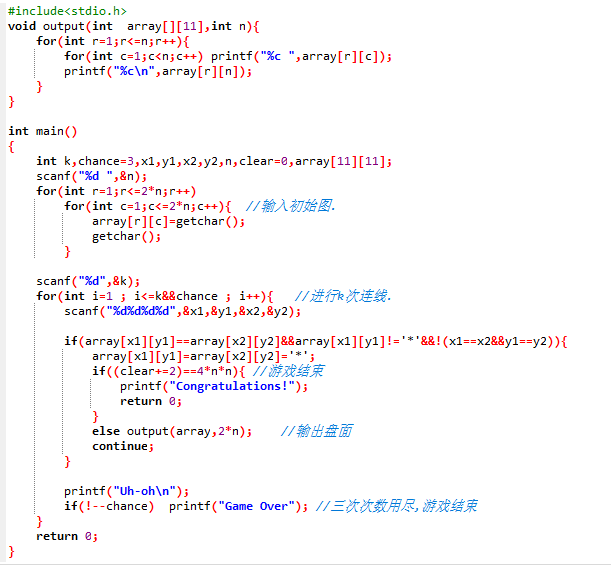### 4.本题调试过程碰到问题及PTA提交列表情况说明。

• 一开始没考虑已被消除的字母之间的连线,而且以为k可以多次输入.补齐条件后解决问题.

## 题目3：出生年

### 1. 本题PTA提交列表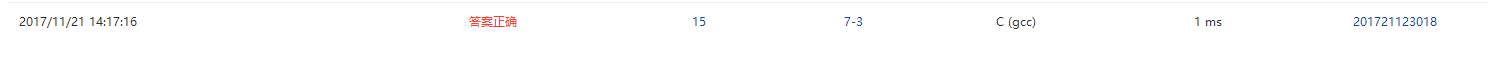### 2.设计思路

int diff(int a[],int year):

for(i=3 ; year ; year/=10) a[i--]=year%10;
for i=1 to 3
定义flag=1标识该数是否为不同数.
for i=0 to i-1
如果a[u]==a[i] flag=0
end for
如果flag==1 count++
end


main():
(int)定义birth表示出生年,year表示目标年,n表示不同数数量a={0,0,0,0}储存年份中各位数的数值.

year=birth
loop
++year
until count==n



### 3.代码截图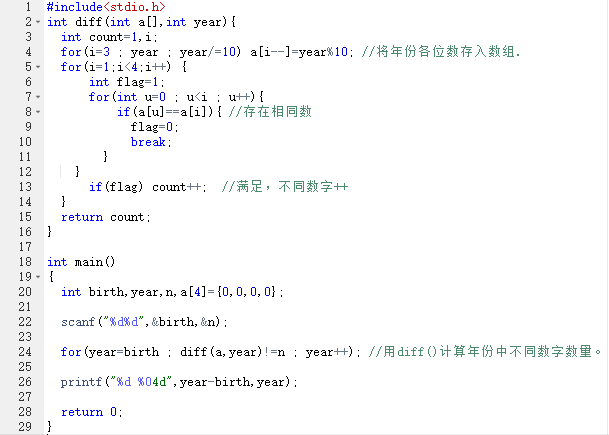• 无问题

# 二、截图本周题目集的PTA最后排名。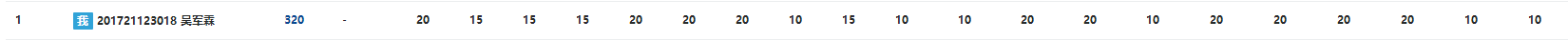# 三、同学代码结对互评

林怡鹏的代码

int main(){
int n,i,j;
scanf("%d",&n);
int a[n],b[n],c[n];
for(i=0;i<n;i++){
scanf("%d",&a[i]);
}
for(i=0;i<n;i++) b[i]=100;
for(i=0;i<n;i++) c[i]=0;
for(i=0;i<n;i++) {
printf("%d ",a[i]);
for(j=0;j<=i;j++){
if(a[i]<=b[j]){
b[j]-=a[i];
printf("%d\n",j+1);
c[i]=j+1;
break;
}
}
}
int max=c;
for(i=1;i<n;i++){
if(c[i]>max)
max=c[i];
}
printf("%d",max);
return 0;
}

我的代码

#include<stdio.h>
int main()
{
int n,weight,index,max=0,box;
scanf("%d",&n);
for(int i=1;i<=n;i++){
box[i]=100; //初始容量为100
scanf("%d",&weight);
for(index=1;;++index){
if(box[index]>=weight ){
box[index]-=weight;
if(index>max) max=index;
printf("%d %d\n",weight,index);
break;
}
}
}
printf("%d",max);
return 0;
}

• 林怡鹏的代码用数组a[]记录每次装箱的重量,b[]表示箱子剩余容量,c[]记录每次装箱所用的箱子的序号,这样可以分开处理各个要求,便于求解.
• 我的代码将箱子大小的初始,装箱及求箱子最大序号放在一起,比较简洁.
• 我更喜欢我的代码

# 四、本周学习总结

## 1.你学会了什么？

### 1.2 字符串的结束标志是什么，为什么要结束标志？

• 结束符为‘\0’
• 可以用结束标志判断什么时候停止输出。

### 1.3 字符串输入有哪几种方法？

• 1.用for循环将字符一个一个存入数组。
• 2.用gets()直接输入以回车作为结束标识的字符串。
• 3.用scanf("%s“,数组名)输入以空格，回车，制表符为结束标识的字符串。

### 1.4 数字字符怎么转整数，写个伪代码？

定义num,sum=0储存整数大小,i=0.
gets(num)
loop
sum=sum*10+num[i++]-'0'
until num[i]=='\0'.


### 1.5 十六进制、二进制字符串如何转10进制？写伪代码？

• 2进制转10进制只需将1.4中sum=sum10+num[i++]-'0'改为sum=sum2+num[i++]-'0'
• 16进制转10进制，将1.4中的循环体改为：
如果num[i] >= '0'&&num[i] <= '9'   sum=sum*16 + num[i++]-'0'



• 感觉还是要多打开思路

## 3.期中考试小结

### 3.1 你认为为什么没考好？

• 没复习
• 基础知识不牢固,对拓展知识的了解不足.
• 手写代码速度慢.

### 3.3 下半学期要怎么调整C的学习？

• 多学课外内容提高编程能力,多学习其他技能.
posted on 2017-12-03 22:38  嗡嗡_嗡  阅读(303)  评论(2编辑  收藏  举报